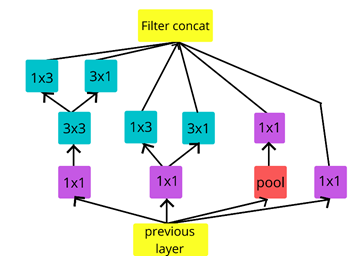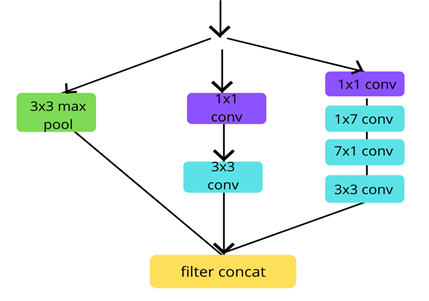top of page
Search

# How to use Inception Model for Image recognition

Have you ever thought of how search engines and things like these work with image processing? If you want to try it by yourself then you’re at the right place, in this blog we will be discussing simple object detection using Inception model.

Topics covered in this Blog are:

• What is Inception.

• Why we use Inception.

• Types of Inception.

• Layer architecture of inception v3.

• How to use Inception v3 for object detection from an Image, Python implementation.

• Conclusion.

What is Inception?

• Inception model is a convolutional neural network which helps in classifying the different types of objects on images.

• It uses ImageNet dataset for training process.

• In the case of Inception, images need to be 299x299x3 pixels size.

• Inception Layer is a combination of 1×1, 3×3 and 5×5 convolutional layer with their output filter banks concatenated into a single output vector forming the input of the next stage.

• And firstly introduced in 2015.

Why we use Inception?So, if the size of the object in the image is of different size so it difficult to detect or fetch the correct information of the object from the various images, then we need to give the correct size of filter. Let us understand this by an example:Fig 1.Fig 2.

Since, here are two different images of same object but with different size, in image 1, the car occupies more region so it requires higher size filters, and in image 2, car occupies less region which requires lower size filter. So therefore, Inception model allows the internal layers to choose which filter size will be relevant to learn the required information, so even if the size of the object is in the image is different, the layers work according to recognize the face.

• Other models generate some computational complexities and overfitting, therefore we use versions of Inception model to reduce such types of problems.

• The model attained greater than 78.1% accuracy in about 170 epochs on each of these.

• While training, the model requires several passes through the training dataset to improve its image recognition proficiency. In the case of Inception v3, depending on the global batch size, the number of epochs needed will be somewhere in the 140 to 200 range.

• File inception_preprocessing.py contains a multi-option pre-processing stage with different levels of complexity that has been used successfully to train Inception v3 to accuracies in the 78.1-78.5% range.

• Both in terms of speed and accuracy, it uses a lot of tricks to push performance. The model itself is made up of symmetric and asymmetric building blocks, including convolutions, average pooling, max pooling, filter concatenations, dropouts, and fully connected layers. Batch normalization is used extensively throughout the model and applied to activation inputs. Loss is computed via SoftMax function.

Types of Inception:

Types of Inception versions covered in this blog are:

• Inception v1

• Inception v2

• Inception v3

1) Inception v1 (Naïve version)

Naïve version performs convolution on an input, with 3 different sizes of filters i.e. 1x1, 3x3 and 5x5 convolution. Furthermore, max pooling is also performed. The output’s layers are then concatenated and passed to the next Inception module. Image of the Naïve Inception module is given below:We know that, deep neural networks are computationally expensive. To make it computationally cheaper, the number of input channels kept limited by adding an extra 1x1 convolution before the 3x3 and 5x5 convolutions. And always remember to put 1x1 convolution after max pool layer, to put it before instead. Though adding an extra operation may seem contradictory, 1x1 convolutions are far cheaper than 5x5 convolutions, and the reduced number of input channels also help.

By using the dimension reduced inception module, a neural network architecture was built. This was popularly known as GoogLeNet (Inception v1). The architecture is shown below:Image source Going deeper with convolutions

GoogLeNet has 9 such inception modules stacked linearly. It is 22 layers deep or 27, including the pooling layers. It uses global average pooling at the end of the last inception module.

As the model is very deep, so it can generate vanishing gradient problem, to prevent the middle part of the network from vanish, the authors introduced two auxiliary classifiers. They essentially applied SoftMax to the outputs of two of the inception modules, and evaluated an auxiliary loss over the same labels.

2) Inception v2

It proposes a number of filters which leads to increases the accuracy and reduces the computational complexity. In this version we use factorization method so that computational complexity becomes less. And by this way we can make our module deeper instead of wider.

• In Inception v2 architecture, 5×5 convolution is replaced by the two 3×3 convolutions. This also decreases computational time and thus increase computational speed because a 5×5 convolution is 2.78 more expensive than 3×3 convolution. So, using two 3×3 layers instead of 5×5 boost the performance of architecture.• This architecture also converts nxn factorization into 1xn and nx1 factorization. As we discuss above that a 3×3 convolution can be converted into 1×3 then followed by 3×1 convolution which is 33% cheaper in terms of computational complexity as compared to single 3×3 convolution.• To deal with the problem of the representational bottleneck, the feature banks of the module were expanded instead of making it deeper. This would prevent the loss of information that causes when we make it deeper.3) Inception v3

They argued that they function as regularizes, especially if they have Batch Norm or Dropout operations because authors noted that the auxiliary classifiers didn’t contribute much until near the end of the training process when accuracies were nearing saturation.

So, version 3 adds some of the following modifications:

• Factorized 7x7 convolutions.

• Batch Normalization in the Auxiliary Classifiers (to overcome the problem of vanishing gradient).

• Use of RMSprop optimizer.

• Asymmetric convolutions.

• Smaller convolutions.

• Grid size reduction.

• Label Smoothing (A type of regularizing component added to the loss formula that prevents the network from becoming too confident about a class. Prevents overfitting).

Some points to be noted:

• this version has network of 48 layers,

• trained on some part of ImageNet dataset,

• and in 1000 different classes.

Now, before going towards the main code, let’s have a brief lookout about the model we’ll be using. A little knowledge of the model will greatly help you in understanding coding afterwards:

Layer Architecture of Inception v3:Now you must be wondering of what are these Inception A-B-C and Reduction A-B, let’s give it a look at these architectures also:

1) Inception A2) Reduction A3) Inception B4) Reduction B5) Inception CSo, now you're all prepared to do some coding. So, let's get started and create a simple code for simple object detection.

### How to use Inception V3 for object detection from an Image:

Python Implementation:

```from keras.applications import InceptionV3
from keras.applications import imagenet_utils
from keras.applications.inception_v3 import preprocess_input
import numpy as np
import cv2

img_path = 'C:/Users/HP/Desktop/obj/peacock.jpg'

#resize the image to 299x299 square shape
img = img.resize((299,299))
#convert the image to array
img_array = img_to_array(img)

#convert the image into a 4 dimensional Tensor
#convert from (height, width, channels), (batchsize, height, width, channels)
img_array = np.expand_dims(img_array, axis=0)

#preprocess the input image array
img_array = preprocess_input(img_array)

#Load the model from internet / computer
#approximately 96 MB
pretrained_model = InceptionV3(weights="imagenet")

#predict using predict() method
prediction = pretrained_model.predict(img_array)
#decode the prediction
actual_prediction = imagenet_utils.decode_predictions(prediction)

print("predicted object is:")
print(actual_prediction)
print("with accuracy")
print(actual_prediction*100)

#display image and the prediction text over it
#display prediction text over the image
cv2.putText(disp_img, actual_prediction, (20,20), cv2.FONT_HERSHEY_TRIPLEX , 0.8, (255,255,255))

#show the image
cv2.imshow("Prediction",disp_img)
cv2.waitKey(0)

```

Outputs: detected objectsDetection 1Detection 2Detection 3

Here we can see that all the object are detected by the model correctly, but with pretty lower accuracy as we can see it while running the program.

Some unwanted predictions:Fig 1.Fig 2.

Here, in these images, we want to detect the whole image for car and tree, but this time it detects another object present in the image, so we can say that we have to insert some more specific information about the region of object that we want. Thus, this is one the problem we can face while using this model.

Conclusion:

• Efficient utilization of computing resource with minimal computational cost.

• Ability to extract features from input data at varying scales through the utilization of varying convolutional filter sizes.

• Faster and more accurate than other previous pretrained models.

Limitations:

Complexity, so to reduce the complexity of the model, Inception v4 and models after that were introduced by the authors.

Thank you everyone for reading this blog, hope now you are pretty familiar with this concept. Happy learning!

References:

 C. Szegedy, W. Liu, Y. Jia, P. Sermanet, S. Reed, D. Anguelov, D. Erhan, V. Vanhoucke, and A. Rabinovich. Going deeper with convolutions. In Proceedings of the IEEE Conference on Computer Vision and Pattern Recognition, pages 1–9, 2015.

 C. Szegedy, Vincent Vanhoucke, Sergey Ioffe sioffe, Jonathon Shlens, Zbigniew Wojna University College London. Rethinking the Inception Architecture for Computer Vision, Dec 2015.